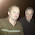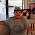13 Apr 2013

The op-amp as a comparator : temperature change detection circuit

In this post, we're going to take a brief look at one way of using an operational amplifier. To test the op-amp as a comparator, we will build a small and simple working circuit. Before diving in, let's take a moment to discuss about the operational amplifier.

Operational amplifier theory

The operational amplifier is a type of amplifier that can be use in a lot of different arrangements to create filters, comparators, integrators, inverting/non-inverting amplifiers etc... In the picture below, you can see the schematic representation of an op-amp and it's different terminals.

As you can see, it needs a positive and negative power supply. The op-amp has an electrical caracteristic called the open-loop gain. This gain is really high and usually range from 100,000@1,000,000. In open-loop, the relation between the output and inputs is dictated by this formula :

It is important to remember that the voltage on the output pin can only swing between the positive and negative supply voltage.

Op-amps are packaged into chips. It's possible that there are multiple op-amps in the same chip. Here is the pinout of the CA3140 IC :

Comparator mode

The principle of operation of the comparator mode is quite simple. In the equation, if the result of the substraction in the parenthesis is positive, the output will equal the positive supply (because of the high open-loop gain). If it's negative, the output will be limited to the negative supply, ground in most of applications. Briefly said (with a LED connected at the output) if V+ is at an higher voltage than V- the LED will be on.

Single supply (rail-to-rail) vs. dual supply

Most op-amp cannot drive it's output signal to their supply, only close to. If you want the output to go completely from a supply to the other, you need to order a rail-to-rail op-amp. These ones can be a little bit pricier. Let's take a look at the internal of a cheap dual supply op-amp that I purchased from Tayda Electronics.

I highlighted in red the components that limit the output pin from going all the way to the supply pins voltage. In the upper part, you have a diode (D7) with a forward drop of 0.7 volt and a transistor (Q18) that will saturate at 0.2-0.3 volt. This means that we cannot go higher than about 1 volt below the positive supply rail. For the negative supply, the transistors (Q15&Q16) will limit the voltage from going lower than 0.2-0.3 volt if the negative pin is tied to ground. By example, if we connect an op-amp to a 12 volt supply source, it's outuput will swing from 0.3 to 11 volts.

The temperature detect circuit

To demonstrate the comparator mode of an operational amplifier, I made a little circuit that use a thermistor. It detects a change of temperature above the ambient room temperature. Take a look at the schematic :

The thermistor is a resistance that is varying with the temperature. At the room temperature, the measured resistance is about 10kΩ, but when I touch it with my finger, it rapidly drop to 7kΩ. The 10kΩ fixed resistor in series with the thermistor make a voltage divider.

At ambient temperature, there is 6 volts at the voltage divider but when I touch the thermistor, the voltage goes a little bit below 5 volts. The output of the voltage divider is brought to the inverting input (-) of the op-amp. I want to compare this to 5 volts, so I used a 5V voltage regulator at the non-inverting input (+) (I was too lazy to make a 5V voltage divider from resistors). When I touch the thermistor with my finger, the (+) pin voltage is above the (-) pin voltage so the output goes almost to the positive supply. This cause the LED to light. (I changed the LED for a green one because the other one was too bright for the camera)

Conclusion

The principle of operation of this circuit can be transposed to a lot of different sensors or output device. It could have been a photoresistor (light detector) in place of the thermistor. We also could have replaced the LED by a fan so it could cool a hot device. Except for using an operational amplifier as a comparator, we rarely use it in open-loop (without any feedback). In a few articles, we'll take a look at other useful examples of circuits that uses an op-amp.

1.2.